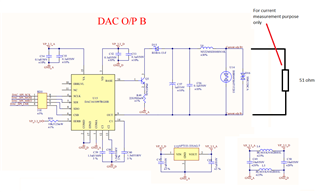# DAC161S997: DAC is not giving required current at output

Part Number: DAC161S997

Hi,

We are using DAC161S997RGHR in our 4-20mA converter application. We have designed the schematic as per datasheet but we are not getting current output. We have used 51 ohm resistor across DAC output to measure current. As per ohms law we should get 1V across this resistor when current is 20mA and 200mV when current is 4mA. But We are constantly getting 0.85V across this resistor irrespective of any data on SPI bus.

Attached is our schematic. Can you please review and let us know if we are doing it correct.

VP_3.3_A and VP_3.3_D are locally supplied (self powered) and only the current loop is loop powered. Do we need to send GND_D to loop receiver?

Regards,
Ramesh Shinde• Ramesh,

The loop power should be isolated from the DAC161S997 power. So you shouldn't send the GND_D to the loop. Often, I refer back to Figure 16 in the datasheet. I would post that here, but I'm having a hard time inserting images into E2E for now. Pull up the datasheet, and I'll refer to that figure.

The LOOP supply ground (OUT,LOOP-) must be floating with respect the the device ground (GND, COMA). In this way, OUT will be allowed to drop slightly below COMA. Note that the voltage difference will be R2*I2. R2 is the internal 40Ω resistor and I2 basically is the loop current (really the loop current is IE+IDAC which is close to IE).

Looking at your schematic, you have analog and digital grounds and analog and digital supplies. I would remove the inductors and use just a single supply and single ground. That would simplify your schematic. Then I would start making some measurements with a precision multimeter to figure out where your current is going.

In particular I'd measure the following:
Voltage from BASE to GND_A
Voltage across the emitter resistor
Voltage from OUT to GND_A
Voltage from LOOP+ to GND_A

I'd also check the VCE voltage of Q3 and the voltage drop across D15 just to be sure.

Joseph Wu